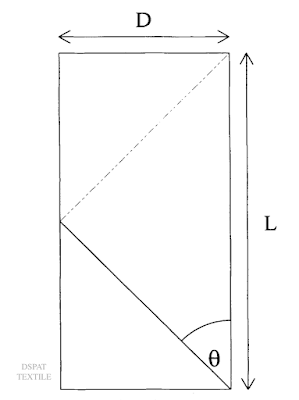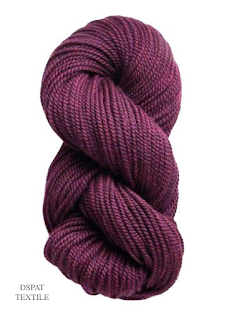# Twist Angle

The twist angle (𝛉) is the angle between a tangent to the helix formed by the fibre and yarn axis due to twist insertion. It grows with the twist.

By unrolling the surface layer, it converts into a square, and from there it is clear that the fibres become the hypotenuse of a right-angled triangle.

Suppose the yarn diameter is D and the fibre takes one full turn of twist in a length of yarn L. The fibre makes an angle with the yarn axis is 𝛉.

Therefore, tan 𝛉 =  𝝿 D/LTwist angle

The greater the diameter of the yarn, the greater the angle of twist (for the same twist level).

As 1/L is equivalent to turns per unit length then:

tan 𝛉 D * turns/unit length.

In the indirect system for measuring linear density, the diameter is proportional to 1/√ count.

Therefore, tan  𝛉 (turns/unit length) / √ count.

The twist factor is defined using this relationship:

Turns per unit length = K * √ count.

K = (turns/unit length) / √ count

Here K is the twist factor.

The value of K differs with each counting system.

(a) In the case of Tex (direct system): K = TPM x √ count

(b) For indirect: K = TPI (or TPM or TCM) / √ count

(Value of K ranges 3.0-8.0 from softer to harder)

Consider Count in terms of Ne c,

tan𝛉 = (𝝿 * turns per unit length) / (28 * √ count).

Therefore, Turns per unit length = (28 * tan𝛉 * √ count) / 𝝿

Turns per unit length = Twist Multiplayer (K) * √ count

Where, Twist Multiplayer K = 28tan𝛉 / 𝝿.

### Twist effects on yarn and fabric

properties:

(a) Handle - As the twist level in a yarn is increased it becomes more compact because the fibres are held more tightly together, so giving a harder feel to the yarn.

Because of the decrease in the yarn diameter, its covering power is reduced. A fabric made from a high-twist yarn will therefore feel harder and will also be thinner.

A fabric produced from a low-twist yarn will have a soft handle but at the same time, weaker yarn thus resulting in pilling and low abrasion resistance of fabric.

(b) Moisture absorption - High twist holds the fibres tight thus restricting water to enter a high twist yarn is used where a high degree of water repellency is required, e.g. in gabardine fabric. Low-twist yarn is used where absorbency is required.

(c) Wearing properties - With an increase in twist level wearing properties (abrasion and pilling) are improved.

A high level of twist helps to resist abrasion as the fibres can't easily pull out of the yarn. The same effect also helps to prevent pilling (which results from the entanglement of protruding fibres).

(d) Aesthetic effects - The level of twist in yarn alters its appearance both by changing the thickness and light-reflecting properties. Different patterns can be produced in a fabric by using similar yarns but with different twist levels; a shadow stripe can be produced by weaving alternate bands of S and Z twist yarns.

The level of twist can also be used to enhance or subdue a twill effect: a Z-twill fabric produced by weaving Z-twist yarns will have an enhanced Z-twill effect. The same is the case for S-twill.

(d) Faults - Because the level of twist in yarn can change its diameter and other properties such as absorption; the same yarn can change the appearance of fabric, so giving rise to complaints.

Twist Applications: Georgette is made of highly twisted yarn (up to 1000 TPM) by weaving S and Z twisted yarns alternately both in warp and weft direction.

Chiffon is made in the same way but the yarn is more twisted (up to 2000 TPM) and finer than that used in georgette - Cuprammonium rayon is used.

Herringbone is made by using yarns of different types and levels of twists. The twist is usually expressed as the number of turns per unit length, e.g. TPMPly twisted yarn

Questions -

1. What twist angle is in yarn?
2. How twist angle is measured?
3. What are the effects of twists on yarn and fabric? OR which properties of yarn and fabric are affected by changes in twist angle?

References

Lord, P. R. (2003). Handbook of yarn production: Technology, science and economics (1st ed.). Woodhead Publishing.

NPTEL IITm. (n.d.). Nptel.Ac.In. from https://nptel.ac.in/courses/116102029

Saville, B. P. (1999). Physical testing of textiles. Woodhead Publishing.

Testometric co. Ltd. (n.d.). Testometric.co.uk. from https://www.testometric.co.uk/

Yarn tests. (2019, July 23). ATIRA; Ahmedabad Textile Industry’s Research Association. https://atira.in/yarn-tests/

Sobuj, M. S. R. (2015, June 7). Twist measurement in yarn. Textile Study Center. https://textilestudycenter.com/twist-measurement-in-yarn/

Yarn Twist Tester. (n.d.). Labtechcn.com. from https://www.labtechcn.com/yarn-twist-tester.html

(Part-1) Yarn twist introduction

(Part-2) Twist angle and its effect

(Part-3) Yarn twist measurement - direct method

(Part-4) Yarn twist measurement - continuous untwist method

(Part-5) Yarn twist measurement - Untwist - twist method or twist contraction method

Writer -
DSPAT Team

Name

Email *

Message *